# Venn diagram

University students chose a foreign language for the 1st year. Of the 120 enrolled students, 75 chose English, 65 German, and 40 both English and German. Using the Venn diagram, determine:
- how many of the enrolled students chose English only
- how many of the enrolled students chose only German
- How many of the enrolled students chose English or German
- How many of the enrolled students chose neither English nor German

a =  35
n =  25
c =  100
d =  20

### Step-by-step explanation:

$n=65-p=65-40=25$
$c=a+n+p=35+25+40=100$
$d=120-c=120-100=20$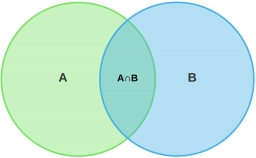Did you find an error or inaccuracy? Feel free to write us. Thank you!## Related math problems and questions:

• Language courses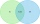Of the company's 60 employees, 28 attend an English course, 17 take a German course, and 20 do not attend any of these courses. How many employees attend both courses?
• Christmas or EasterPlease calculate this example by the Venn equation. They asked 73 students whether they like Christmas or Easter. 34 of them like one of the holidays. 39 loves Easter. There are twice as many students who wish both holidays as those who only love Easter.
• There 11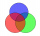There are 50 pupil in the class. Out of of this number,1/10 speak French only and 4/5 of the 10 remainder speak both French and English . If the rest speak English only find the number of students who speak only English?
• 80 students80 students were asked what type of test they preferred. 50 students said they liked multiple choice and 42 liked true or false. If 36 liked both multiple choice and true or false types, how many students preferred multiple choice only?
• RemaindersIt is given a set of numbers { 170; 244; 299; 333; 351; 391; 423; 644 }. Divide this numbers by number 66 and determine set of remainders. As result write sum of this remainders.
• MarketingYear; money spent on advertising; profit 2008 2 12 2009 5 20 2010 7 25 2011 11 26 2012 15 40 1. draw a scatter diagram depicting the data. 2. calculate the Pearson's correlation coefficient. 3. determine the linear regression equation.
• SalamiHow many ways can we choose 5 pcs of salami if we have 6 types of salami for 10 pieces and one type for 4 pieces?
• News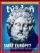From 28 students buy magazine 10 The economist students, 10 Pravda students and 18 students don't buy any of these magazines. How many students buy both magazines?
• The rawThe raw data presented here are the scores (out of 100 marks) of a market survey regarding the acceptability of new product launched by a company for random sample of 50 respondents: 40 45 41 45 45 30 30 8 48 25 26 9 23 24 26 29 8 40 41 42 39 35 18 25 35
• English and math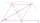1/5 of a class study English, and 3/5 study mathematics. If the class consist of 80 students, how many students study English and mathematics in the class?
• SymbolsIf 2*3 = 60 ; 3*4 = 120 and 4*5 = 200, what is 2*5?
• Brothers and sisters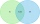There are 35 children in the class, 23 of them have a brother, and 27 of them have a sister. How many children have both a brother and a sister when there are 5 children in the class who have no brother or sister?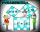Two fifth-graders teams competing in math competitions - in Mathematical Olympiad and Pytagoriade. Of the 33 students competed in at least one of the contest 22 students. Students who competed only in Pytagoriade were twice more than those who just competSuppose Set B contains 69 elements and the total number elements in either Set A or Set B is 124. If the Sets A and B have 29 elements in common, how many elements are contained in set A?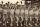Athletes standing in rows of eight. Ivan estimated that athletes are more than 120. Peter claimed that there are fewer than 130. Both are right. How many are athletes?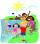Out of the 180 students at a summer camp, 72 signed up for canoeing. Twenty-three students signed up for trekking, and 13 of those students also signed up for canoeing. Use a two-way table to organize the information and answer the following question: AppThere are 27 pupils in the classroom. They can swim 21 and ski nine pupils. Neither swim nor ski three pupils. How many pupils can swim and ski?